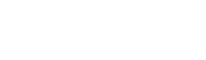# Coding the Fibonacci sequence using Python’s recursive functions

スポンサーリンク

Hello, I’m Yuki, a student engineer.

If you are an aspiring engineer, you are probably often asked about the Fibonacci sequence in coding tests.

This time, I would like to code the Fibonacci sequence, which is also often asked in coding tests.

スポンサーリンク

## Definition of the Fibonacci Sequence

Let us output the Fibonacci sequence that satisfies the following conditions.

f(0) = 0

f(1) = 1

f(n) = f(n-1) + f(n-2)

## Tips of coding

Asymptotic expressions are used for sequences of numbers, so it seems possible to use an asymptotic expression

Use the map function to output the return value of a function in order

## Coding

Here is the Fibonacci function implemented in code.

def fib(n):
if n <= 1:
return n

return fib(n-1) + fib(n-2)

The Fibonacci sequence will be output up to 10 terms.

for i in map(fib, range(10)):
print(i)

Output:

0
1
1
2
3
5
8
13
21
34

## At the end

There are many other methods besides the one presented here.

From the standpoint of being more concise and using less computation, the code introduced here is not necessarily the best.

Since simplicity was the key to the code presented here, it would be good to try to write a code that can reduce the amount of calculation.

Yuki